# Archive | How to with JMP## Attribute MSA with JMP

Use JMP to Implement an Attribute MSA Data File: “AttributeMSA.jmp” Steps in JMP to run an attribute MSA: Click Analyze -> Quality & Process ->Variability/Attribute Gauge Chart Select “Appraiser A”, “Appraiser B” and “Appraiser C” as “Y, Response” Select “Part” as “X,Grouping” Select “Reference” as “Standard” Select “Attribute” as the “Chart Type” Click “OK” Click on […]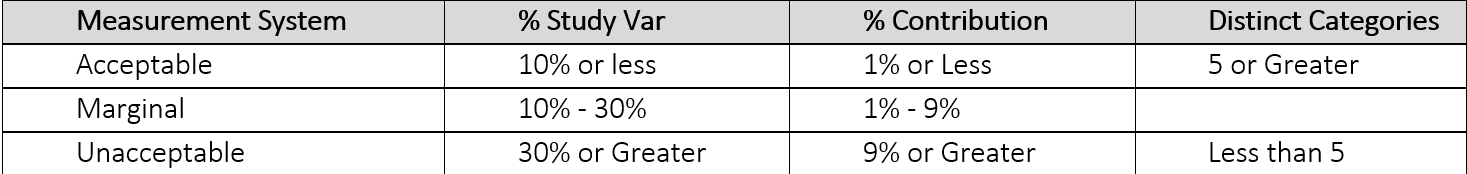## Variable Gage R&R with JMP

What is Variable Gage R&R? Variable Gage Repeatability & Reproducibility  (Gage R&R) is a method used to analyze the variability of a measurement system by partitioning the variation of the measurements using ANOVA (Analysis of Variance). Whenever something is measured repeatedly or by different people or processes, the results of the measurements will vary. Variation […]## Scatter Plot with JMP

What is a Scatter Plot? A scatter plot is a diagram to present the relationship between two variables of a data set. A scatterplot consists of a set of data points. On the scatterplot, a single observation is presented by a data point with its horizontal position equal to the value of one variable and […]## Histogram Rendering with JMP

What is a Histogram? A histogram is a graphical tool to present the distribution of the data. The X axis of a histogram represents the possible values of the variable and the Y axis represents the frequency of the value occurring. A histogram consists of adjacent rectangles erected over intervals with heights equal to the […]## Box Plot with JMP

What is a Box Plot? A box plot is a graphical method to summarize a data set by visualizing the minimum value, 25th percentile, median, 75th percentile, the maximum value, and potential outliers. A percentile is the value below which a certain percentage of data fall. For example, if 75% of the observations have values […]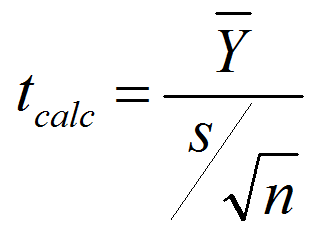## One Sample t Test with JMP

One Sample t Test What is a t Test? In statistics, a t test is a hypothesis test in which the test statistic follows a Student’s t distribution if the null hypothesis is true. We apply a one sample t test when the population variance (σ) is unknown and we use the sample standard deviation […]## Central Limit Theorem with JMP

What is Central Limit Theorem? The Central Limit Theorem is one of the fundamental theorems of probability theory. It states a condition under which the mean of a large number of independent and identically-distributed random variables, each of which has a finite mean and variance, would be approximately normally distributed. Let us assume Y1, Y2 […]## Multi Vari Analysis with JMP

What is Multi-Vari Analysis? Multi vari analysis is a graphic-driven method to analyze the effects of categorical inputs on a continuous output. It studies how the variation in the output changes across different inputs and helps us quantitatively determine the major source of variability in the output. Multi-vari charts are used to visualize the source […]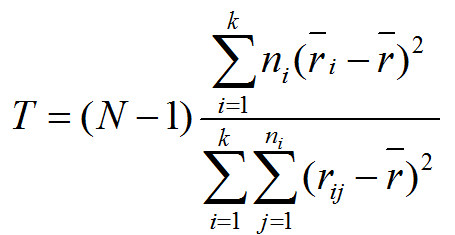## Kruskal Wallis with JMP

What is Kruskal–Wallis One-Way Analysis of Variance? The Kruskal Wallis one-way analysis of variance is a statistical hypothesis test to compare the medians among more than two groups. It is an extension of Mann–Whitney test. While the Mann–Whitney test allows us to compare the samples of two populations, the Kruskal Wallis test allows us to […]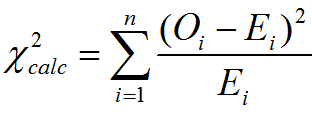## Chi Square Test with JMP

Chi Square (Contingency Tables) We have looked at hypothesis tests to analyze the proportion of one population vs. a specified value, and the proportions of two populations, but what do we do if we want to analyze more than two populations? A chi-square test is a hypothesis test in which the sampling distribution of the […]## Correlation Coefficient with JMP

What is Correlation? Correlation is a statistical technique that describes whether and how strongly two or more variables are related. Correlation analysis helps to understand the direction and degree of association between variables, and it suggests whether one variable can be used to predict another. Of the different metrics to measure correlation, Pearson’s correlation coefficient [...]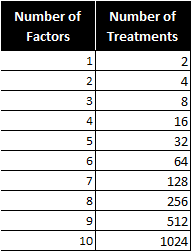## Fractional Factorial Designs with JMP

What Are Fractional Factorial Experiments? In simple terms, a fractional factorial experiment is a subset of a full factorial experiment. Fractional factorials use fewer treatment combinations and runs Fractional factorials are less able to determine effects because of fewer degrees of freedom available to evaluate higher order interactions Fractional factorials can be used to screen [...]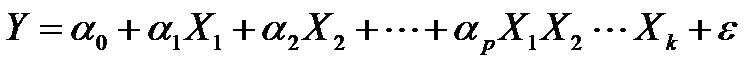## Full Factorial DOE with JMP

Full Factorial DOE In a full factorial experiment, all of the possible combinations of factors and levels are created and tested. For example, for two-level design (i.e.each factor has two levels) with k factors, there are 2k possible scenarios or treatments. Two factors, each with two levels, we have 22 = 4 treatments Three factors, each [...]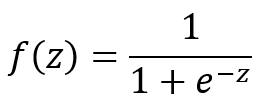## Logistic Regression with JMP

What is Logistic Regression? Logistic regression is a statistical method to predict the probability of an event occurring by fitting the data to a logistic curve using logistic function. The regression analysis used for predicting the outcome of a categorical dependent variable, based on one or more predictor variables. The logistic function used to model [...]## Stepwise Regression with JMP

What is Stepwise Regression? Stepwise regression is a statistical method to automatically select regression models with the best sets of predictive variables from a large set of potential variables. There are different statistical methods used in stepwise regression to evaluate the potential variables in the model: F-test T-test R-square AIC Three Approaches to Stepwise Regression [...]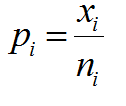## P Chart with JMP

What is a P Chart? The P chart plots the percentage of defectives in one subgroup as a data point. It considers the situation when the subgroup size of inspected units is not constant. The underlying distribution of the P-chart is binomial distribution. P Chart Equations Data Point:         Center Line:   [...]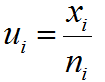## U Chart with JMP

What is a U Chart? The U chart is a type of control chart used to monitor discrete (count) data where the sample size is greater than one, typically the average number of defects per unit. Defect vs. Defective Remember the difference between defect and defective? A defect of a unit is the unit’s characteristic [...]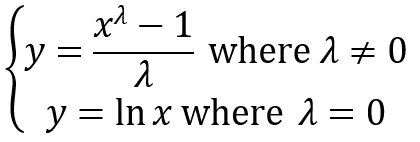## Box Cox Transformation with JMP

What is a Box Cox Transformation? Data transforms are usually applied so that the data appear to more closely meet assumptions of a statistical inference model to be applied or to improve the interpret-ability or appearance of graphs. Power transformation is a class of transformation functions that raise the response to some power. For example, a [...]